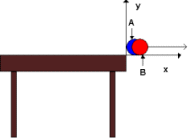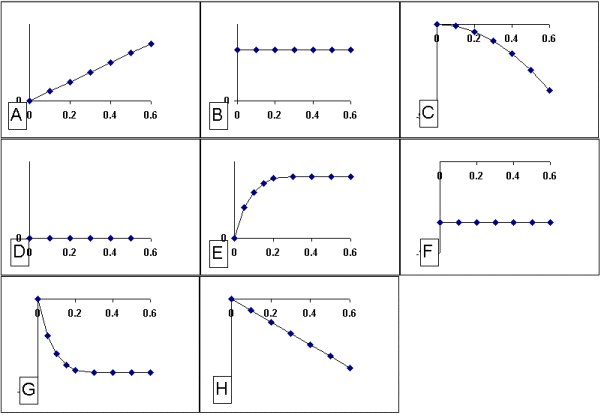Teaching Physics with the Physics Suite Edward F. Redish

Billiards over the edge

 Two identical billiard balls are labeled A and B. Maryland Fats places ball A at the very edge of the table, ball B at the other side. He strikes ball B with his cue so that it flies across the table and off the edge. As it passes A, it just touches ball A lightly, knocking it off. The balls are shown just at the instant they have left the table. Ball B is moving with a speed v0, ball A is essentially at rest. (a) Which ball do you think will hit the ground first? Explain your reasons for thinking so.Below are shown a number of graphs of a quantity versus time. For each of the items below, select which graph could be a plot of that quantity vs. time. If none of the graphs are possible, write N. The time axes are taken to have t=0 at the instant both balls leave the table. Use the x and y axes shown in the figure. For each of the following, which graph could represent:

 (b) the x-component of the velocity of ball B? (c) the y-component of the velocity of ball A? (d) the y-component of the acceleration of ball A? (e) the y-component of the force on ball B? (f) the y-component of the force on ball A? (g) the x-component of the velocity of ball A? (h) the y-component of the acceleration of ball B?Not finding what you wanted? Check the Site Map for more information.